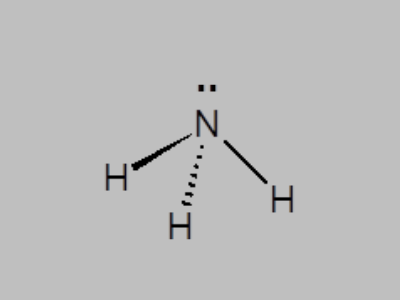# Ammonia Formula

Ammonia formula also referred to as nitrogen trihydride formula or azane formula is one of the most popular formulas in chemistry. Besides, Ammonia is one topic that students come across frequently when studying chemistry. Ammonia is basically a colourless inorganic or alkaline gas having a distinct pungent odour. The compound ammonia is further an important source of nitrogen for many applications in chemical and industrial processes.

## Ammonia Chemical Formula

The molecular formula is derived from the chemical structure of ammonia where the ammonia molecule exhibits a trigonal pyramidal shape with nitrogen attached to the three hydrogen atoms. The nitrogen atom, on the other hand, has a lone electron pair. Ammonia formula is given as NH3.

The key details of ammonia are given below:

 IUPAC ID Azane Formula NH3 or H3N Molar Mass 17.031 g/mol Boiling Point -33.35 °C Melting Point -77.7 °C Density 0.73 kg/m³

### Ammonia Structural Formula

Below students will find the structural formula of ammonia.Keep visiting BYJU’S to learn more about the formulas of different chemical compounds.